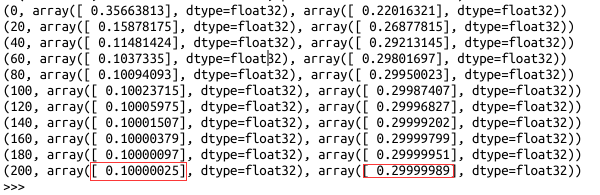# TF Boys (TensorFlow Boys ) 养成记（一）：TensorFlow 基本操作

import tensorflow as tf
hello = tf.constant('Hello TensorFlow!')
sess = tf.Session()
print(sess.run(hello))
a = tf.constant(10)
b = tf.constant(32)
print(sess.run(a + b))

1. 使用图(graphs)来表示计算；

2.在会话(Session)中执行图；

3.使用张量(tensors)来代表数据；

4.通过变量(variables)来维护状态；

5.使用供给(feeds)和取回(fetches)来传入或者传出数据。

import tensorflow as tf
import numpy as np
import os
os.environ['CUDA_VISIBLE_DEVICES']='0'
config = tf.ConfigProto()
config.gpu_options.per_process_gpu_memory_fraction=0.2
sess = tf.InteractiveSession(config=config)

x_data = np.random.rand(100).astype("float32")
y_data = x_data * 0.1 + 0.3

W = tf.Variable(tf.random_uniform(,-1.0,1.0))
b = tf.Variable(tf.zeros())
y = W * x_data + b
loss = tf.reduce_mean(tf.square(y - y_data))
train = optimizer.minimize(loss)
init = tf.initialize_all_variables()
sess.run(init)
for step in xrange(201):
sess.run(train)
if step % 20 ==0:
print(step, sess.run(W), sess.run(b))MNIST手写数字识别在TensorFlow的example中有自带的代码来实现，官方文档也给出了很好的解释，这两个，建议都看，加强自己对TensorFlow的理解。

posted @ 2016-12-19 12:22  Charles-Wan  阅读(9019)  评论(2编辑  收藏